# Theory of Computation questions and answers

## Theory of Computation questions and answers

(1) From the options given below, the pair having different expressive power is

(A) Deterministic Push Down Automata (DPDA) and Non-deterministic Push Down Automata (NPDA)
(B) Deterministic Finite Automata (DFA) and Non-deterministic Finite Automata(NFA)
(C) Single tape turning machine and multi tape turning machine.
(D) Deterministic single tape turning machine and Non-Deterministic single tape turning machine

ANSWER: Deterministic Push Down Automata (DPDA) and Non-deterministic Push Down Automata (NPDA)

(2) The problem that is undecidable -

(A) Finiteness problem for FSA’s
(B) Membership problem for CFG’s
(C) Equivalence problem for FSA’s
(D) Ambiguity problem for CFG’s

(3) The language which is generated by the grammar S-> aSa I bSb I a I b over the alphabet {a, b} is the set of

(A) Strings that begin and end with the same symbol
(B) All odd and even length palindromes
(C) All odd length palindromes
(D) All even length palindromes

(4) Two persons X and Y have been asked to show that a certain problem p is NP-complete. X shows a polynomial time reduction from the 3-SAT problem to p and Y shows a polynomial time reduction from p to 3-SAT. From these reduction it can be inferred that

(A) π is NP-complete
(B) π is NP-hard but not NP-complete
(C) π is in NP but not NP-complete
(D) π is neither NP-hard nor in NP

(5) Out of the three problems S, Q and R, S is an NP-complete problem and Q and R are the two other problems not known to be in NP. Which one of the following statements is true if Q is polynomial time reducible to S and S is the polynomial time reducible to R?

(A) Q is NP-complete
(B) R is NP-complete
(C) Q is NP-hard
(D) R is NP-hard

(6) From the options given below the statement, which is not necessarily true if X1 is the recursive language and X2 and X3 are the languages that is recursively enumerable but not recursive is

(A) X2 ∩ X1 is recursively enumerable
(B) X2 ∪ X1 is recursively enumerable
(C) X2 – X1 is recursively enumerable
(D) X1 – X3 is recursively enumerable

ANSWER: X1 – X3 is recursively enumerable

(7) For the language {ap I P is a prime}, the statement which hold true is

(A) It is not regular but context free
(B) It is regular but not context free
(C) It is neither regular nor context free, but accepted by a turing machine
(D) It is not accepted by turing machine

ANSWER: It is neither regular nor context free, but accepted by a turing machine

(8) The statement that holds true is

(A) Infinite union of finite sets is regular
(B) The union of two non-regular set is not regular
(C) Every finite subset of a non-regular set is regular
(D) Every subset of a regular set is regular

ANSWER: Every finite subset of a non-regular set is regular

(9) The language described by the regular expression (0+1)*0(0+1)*0(0+1)* over the alphabet {0 1} is the set of

(A) All strings containing at least two 1’s
(B) All strings containing at least two 0’s
(C) All strings that begin and end with either 0’s or 1’s
(D) All strings containing the substring 00

ANSWER: All strings containing at least two 0’s

(10) 3-SAT and 2-SAT problems are

(A) NP-complete and in P respectively
(B) Undecidable and NP-complete
(C) Both NP-complete
(D) Both in P

ANSWER: NP-complete and in P respectively

(11) Which one of the following statement is true?

(A) The intersection of two context free languages is context free
(B) A context free language can always be accepted by a deterministic push down automaton
(C) The union of two context free languages is context free
(D) The complement of a context free language is context free.

ANSWER: The union of two context free languages is context free

(12) Let n be the positive integer constant and L be the language with alphabet {a}. To recognize L the minimum number of states required in a DFA will be

(A) 2k + 1
(B) k + 1
(C) 2n + 1
(D) n + 1

(13) Consider a stack, which is limited to 10 items. The language accepted by a push- down automaton in such stack is best described as

(A) Regular
(B) Deterministic context free
(C) Context free
(D) Recursive

(14) Which one of the following statement is true if L denotes the language generated by the grammar S->0S0/00?

(A) L is not context free
(B) L is regular but not 0+
(C) L = 0+
(D) L is context free but not regular

ANSWER: L is regular but not 0+

(15) Consider the regular expression 0 * (10 *) which is similar to the same set as

(A) 0 + (0 + 10) *
(B) (0 +1) * 10 (0 + 1) *
(C) (1 * 0) * 1*
(D) None of the above

(16) W is any string whose length is n in {0, 1}* and L is the set of all sub-strings of W. The minimum number of states in a non-deterministic finite automaton that accepts L is

(A) n
(B) 2n
(C) n + 1
(D) n - 1

(17) The DFA shown below accepts the set of all strings over {0, 1} that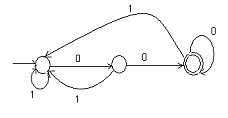(A) End with 00
(B) End with 0
(C) Begin either with 0 or 1
(D) Contain the substring 00

(18) We have two statements S1 and S2 whose definition are as follows:

S1 – {02n In ≥ I} is a regular language.
S2 – {0m 1n 0 1m+n Im=1 and n≥1I is a regular language.

Which one of the following statements is correct?

(A) Both S1 and S2 are correct
(B) Only S2 is correct
(C) Only S1 is correct
(D) Neither S1 nor S2 is correct

(19) Consider a string s over (0+1)*. The number of 0’s in s is denoted by no(s) and the number of 1’s in s is denoted by n1(s). The language that is not regular is

(A) L = {s ∈ (0+1)* I for every prefix s’ of s, I no(s’)-n1(s’) I ≤ 2}
(B) L = {s ∈ (0+1)* I no(s) mod 7 = n1(s) mod 5 = 0}
(C) L = {s ∈ (0+1)* I no(s) is a 3 digit prime}
(D) L = {s ∈ (0+1)* I no(s)-n1(s) I ≤ 4

ANSWER: L = {s ∈ (0+1)* I no(s)-n1(s) I ≤ 4

(20) Which one of the following statement is false?

(A) In Chomsky Normal Form the derivative trees of strings generated by a context-free grammar are always binary trees
(B) If W is the string of a terminals and Y is a non-terminal, the language generated by a context free grammar, all of whose productions are of the form x->W or X->WY is always regular
(C) By using suitable transformation all ε-productions can be removed from any context-free grammar.
(D) Every left recursive grammar can be converted to a right recursive grammar and vice-versa

ANSWER: If W is the string of a terminals and Y is a non-terminal, the language generated by a context free grammar, all of whose productions are of the form x->W or X->WY is

always regular

(21) State table of an FSM is given below. There are two states A And B, one input and one output.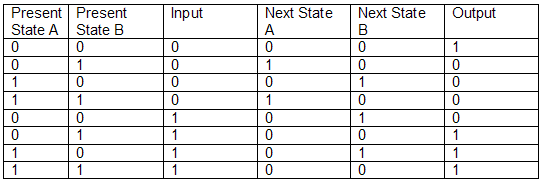Let the initial state be A = 0 and B = 0. To take the machine to the state A = 0 and B = 1 with output = 1 the minimum length of input string required will be

(A) 2
(B) 7
(C) 4
(D) 3

For questions 22 and 23 refer to the data given below:

The figure shown below is a finite state automaton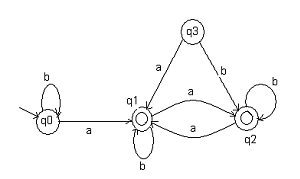(22) Which one of the following is true for this automaton?

(A) b*ab*ab*ab*
(B) b*a(a+b)*
(C) b*ab*ab*
(D) (a+b)*

(23) For the above FSA the equivalent minimum state automaton has the following number of states

(A) 1
(B) 2
(C) 3
(D) 4

(24) Out of the three decision problems P1, P2 and P3, P1 is decidable and P2 is undecidable. The statement that holds true is

(A) P3 is decidable if P3 is reducible to compliment of P2
(B) P3 is decidable if P1 is reducible to P3
(C) P3 is undecidable if P1 is reducible to P3
(D) P3 is undecidable if P2 is reducible to P3

ANSWER: P3 is undecidable if P2 is reducible to P3

(25) G = {S->SS, S->ab, S->ba, S->?} is the context free grammar whose statements are given below:

a. G is ambiguous
b. G produces all strings with equal number of a’s and b’s.
c. Deterministic PDA accepts G

Which of the following statement is true about G?

(A) a, b, c all are true
(B) Only and b are true
(C) Only b and c are true
(D) Only a is true

ANSWER: a, b, c all are true

(26) The minimum number of states in any DFA accepting the regular language L = (111+11111)* is

(A) 5
(B) 7
(C) 9
(D) 11

(27) Consider the language L = {W I W ∈ {0, 1}*, where 0’s and 1’s in W are divisible by 3 and 5 respectively. The minimum state deterministic finite automaton accepting the language L has

(A) 20 states
(B) 5 states
(C) 10 states
(D) 15 states

(28) We have an undirected graph G(V, E) with two problems given below:
α – Does G have an independent set of size IVI – 4?
β – Does G have an independent set of size 5?
The statement that holds true is

(A) α is NP-complete and β is in P
(B) α is in P and β is NP-complete
(C) Both α and β are NP-complete
(D) Both α and β are in P

ANSWER: α is in P and β is NP-complete

(29) Figure shows deterministic finite state automaton M. Let the set of seven bit binary strings whose 1st, 4th and the last bits are 1 is denoted by S. How many strings in S is accepted by M?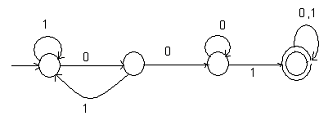(A) 1
(B) 9
(C) 3
(D) 5

(30) Which one of the following statement is true for a regular language L over {a} whose minimal finite state automation has two states?

(A) L must be either {an I n is odd} or {an I n is even}
(B) L must be {an I n is odd}
(C) L must be {an I n is even}
(D) L must be {an I n = 0}

ANSWER: L must be either {an I n is odd} or {an I n is even}

(31) Consider a graph G = (V, E) where I V I is divisible by 3. The problem of finding a Hamiltonian cycle in a graph is denoted by SHAM3 and the problem of determining if a Hamiltonian cycle exits in such graph is denoted by DHAM3. The option, which holds true, is

(A) Only DHAM3 is NP-hard
(B) Only SHAM3 is NP-hard
(C) Both SHAM3 and DHAM3 are NP-hard
(D) Neither SHAM3 nor DHAM3 is NP-hard

ANSWER: Both SHAM3 and DHAM3 are NP-hard

(32) Which one of the following is true for the language {am bn c m+n I m, n≥1}?

(A) It is context-free but not regular
(B) It is regular
(C) It is type-0 but not context-sensitive
(D) It is context-sensitive but not context-free

(33) We have decision problems P1 and P2 as described below:

P1: Does a given finite state machine accept a given string?
P2: Does a given context-free grammar generate an infinite number of strings?

The statement that holds true for P1 and P2 is

(A) Only P2 is decidable
(B) Only P1 is decidable
(C) Neither P1 nor P2 are decidable
(D) Both P1 and P2 are decidable

ANSWER: Both P1 and P2 are decidable

(34) Problem X is given below:

We have a turing machine M over the input alphabet ∑, any state q of M and a word W ∈ ∑*, does the computation of M on W visit the state q? The statement, which holds true

(A) X is undecidable but partially decidable
(B) X is decidable
(C) X is not a decision problem
(D) X is undecidable and not even partially decidable.

ANSWER: X is undecidable but partially decidable

(35) The state diagram describes the finite state machine. A is the starting state and an arc label is x/y where x stands for 1 bit input and y stands for 2 bit output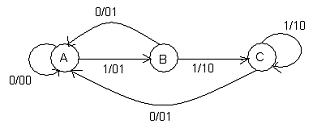(A) Whenever the input sequence is 10 it outputs 00
(B) Whenever the input sequence is 11 it outputs 01
(C) It outputs the sum of the present and the previous bits of the input
(D) None of the above

ANSWER: It outputs the sum of the present and the previous bits of the input

(36) Which one of the following statement is true for the C language?

(A) It is a regular language
(B) It is context-sensitive language
(C) It is context-free language
(D) It is parsable fully only by a turing machine

(37) How many states are present in the smallest finite automaton which accepts the language {x I length of x is divisible by 3}?

(A) 5
(B) 4
(C) 3
(D) 2

(38) The last two symbols of L which is the set of all binary strings are same. In the minimum state deterministic finite state automaton, which is accepting L _____, states are present

(A) 4
(B) 6
(C) 3
(D) 5

(39) The true regular expression is

(A) (r*s*)* = (r+s)*
(B) (r+s)* = r* + s*
(C) r*s* = r* + s*
(D) r(*) = r*

(40) Let n be the length of a character string. How many substrings (of all lengths inclusive) can be formed from n?

(A) n(n-1)/2
(B) n²
(C) (n (n+1)/2) + 1
(D) n

(41) The set which is not countable if we have ∑ = {a, b}, is

(A) Set of all languages over ∑ accepted by turing machine
(B) Set of all regular languages over ∑
(C) Set of all strings over ∑
(D) Set of all languages over ∑

ANSWER: Set of all languages over ∑

(42) How many states are present in the minimum state finite automaton that recognizes the language represented by the regular expression (0+1)(0+1)…..N times?

(A) n+1
(B) n+2
(C) n
(D) 2n

(43) Consider the state table of a finite state machine that has input x and a single output z. The shortest input sequence to reach the final state C if the initial state is unknown is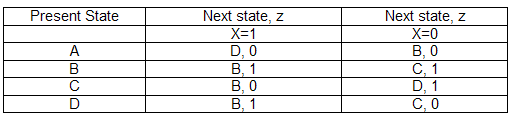(A) 10
(B) 01
(C) 101
(D) 110

(44) The set that can be recognized by a deterministic finite state automaton is

(A) The set {1, 101, 11011, 1110111, …….}
(B) The set of binary string in which the number of 0’s is same as the number of 1’s
(C) 1, 2, 4, 8……2n ….. written in binary
(D) 1, 2, 4, 8……2n ….. written in unary

ANSWER: 1, 2, 4, 8……2n ….. written in binary

(45) Consider the four regular expressions given below;

a. (00)*( ε+0)
b. (00)*
c. 0*
d. 0(00)*

The equivalent regular expression out of the four is

(A) b and c
(B) c and d
(C) a and b
(D) a and c

(46) L1 = Φ and L2 = {a} are the two languages. Out of the following four options the one that represents L1L2* U L1* is

(A) Φ
(B) a*
(C) {ε}
(D) {ε, a}

(47) We have the language L = {ab, aa, baa} and the four strings given below:

I) abaabaaabaa
II) aaaabaaaa
III) baaaaabaaaab
IV) baaaaabaa

The strings present in L* are

(A) I, II and IV
(B) I, II and III
(C) II, III and IV
(D) I, III and IV

(48) Which one of the following is true regarding FOTRAN?

(A) It is a context free language
(B) It is a context sensitive language
(C) It is a regular language
(D) None of the above

ANSWER: It is a context sensitive language

(49) The feature that cannot be captured by context free grammar is

(A) Recursive procedure Syntax
(B) Syntax of if-then-else statement
(C) Arbitrary length of variable names
(D) Variable declared before its use

ANSWER: Variable declared before its use

(50) Which one of the following is applicable for context free languages?

(A) These are closed under union, Kleene closure
(B) These are closed under complement, Kleene closure
(C) These are closed under union, intersection
(D) These are closed under intersection, complement

ANSWER: These are closed under union, Kleene closure

(51) S -> a α b I b a c I ab
S -> α S I b
S -> α bb I ab
S -> bdb I b

The grammar described above is

(A) Context free
(B) Context sensitive
(C) Regular
(D) LR(k)

(52) Match the following

a. Regular expression I. Syntax analysis
b. Pushdown automata II Code generation
c. Dataflow analysis III Lexical analysis
d. Register allocation IV Code optimization

(A) a - III, b - IV, c - I, d - II
(B) a - IV, b - III, c - I, d - II
(C) a - III, b - I, c - IV, d - II
(D) a - II, b - III, c - IV, d - I

ANSWER: a - III, b - I, c - IV, d - II

(53) Which of the following statement is false for a turing machine?

(A) There exists an equivalent deterministic turing machine for every non-deterministic turing machine
(B) Turing decidable languages are closed under intersection and complementation
(C) Turing recognizable languages are closed under union and intersection
(D) Turing recognizable languages are closed under union and complementation

ANSWER: Turing recognizable languages are closed under union and complementation

(54) The problem, which is not NP-hard, is

(A) Finding bi-connected problem of a graph
(B) The graph colouring problem
(C) Hamiltonian circuit problem
(D) The 0/1 Knapsack problem

(55) If P≠NP the statement which holds true is

(A) NP-hard = NP
(B) NP-complete ∩ P = Φ
(C) P=NP-complete
(D) NP-complete=NP

ANSWER: NP-complete ∩ P = Φ

#### Discussion

• RE: Theory of Computation questions and answers -VIVEK VERMA (12/16/17)
• 6th question ka answer aap galat bta rhe ho
• RE: Theory of Computation questions and answers -likitha (08/20/15)
• Can u please give breif descriptions to the problems